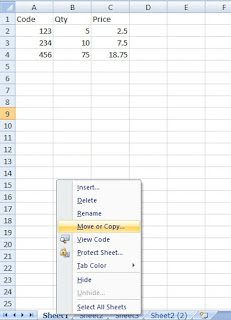# LEARN MS-EXCEL TIPS AND TRICKS

Your Search for Microsoft Excel tips and Tricks ends here.

### Displaying Worksheet Formula

Worksheets can have hundreds of  formulas linked to one another.  Output value of one cell formula can become part of another formula in another cell.  When worksheets of this type involves in complex analysis tasks it becomes difficult to track the relationships between formulas in cells or range of cells, especially when cell references depends on Cell Addresses.  When you insert cells, rows or columns the cell references in formulas can change.

When you start developing something  serious it is a good idea to give unique range-names to Cell or Range of Cells and use those names in expressions.

For example:

Assume that Cell Range B2:B25 contains detailed figures of Income values.  This Range of Cells can be easily referenced with the Range Name Income.  Try the following:

1. Open Microsoft Excel and fill some numeric values in Cells B2 to B25 on Sheet1.
2. Highlight the Cell Range B2:B25.
3. Select Define Name from the Defined Names group from Formulas Menu and type Income as name into the Name control in the displayed dialog box.  The selected Range address will be displayed in the Refers To: control at the bottom of the dialog box as =Sheet1!\$B\$2:\$B\$25.
5. Select Cell B26 and Type the expression =Sum(Income) to get the sum of values from Range: B2:B25.

Note: If you add few rows/cells between B2:B25 and  add values into the added Cells, the Income range will automatically expanded and the correct value will reflect in wherever we use the Income range name.  If you add rows or cells above Cell B2 the Income range will automatically adjust itself to refer to the number of cells within the Income Range to the changed position.

For example: if you have added a single row above the cell B2, the Income Range address B2:B25 will change to B3:B26 to adjust to the changed position of the Income range.

But, if you fill up cells below B25 with values and want to include them into the Income Range you must redefine the Income Range B25:B26 by going through the initial steps that you have followed.

6. Follow the same procedure for defining Range Name for Expenses cell range, after filling up some values into Cells: D2:D25.
7. Now to find the Profit/Loss value the calculation is easy like =Sum(Income) - Sum(Expenses) rather than =Sum(B2:B25)-Sum(D2:D25).  But, the expression =Sum(B2:B25)-Sum(D2:D25) you can write only on the same Workwheet.

You can write the expression =Sum(Income) - Sum(Expenses) in any of your Worksheets within the same Workbook without bothering on which worksheet cell addresses the range name Income refers to.  If you want to refer these ranges, without the range names, on a different Worksheet you must add the Sheet reference to the range in the formula like: =Sum(Sheet1!B2:B25) - Sum(Sheet1!D2:D25).

Always use meaningful names to define Range Names, which have relevance to your task.  This way you will not have any difficulty in memorizing the task related names used in the formula.

Tip:  When you want to take a full view of all the formulas on a particular Worksheet use the Keyboard Shortcut: Ctrl+~ (press and hold Ctrl Key then press the Tilde character (~) key, you will find this key to the left of the numeric key 1.  If you press this Key combination one more time the Worksheet will reset to normal view.  When you are in the Formula View you may take a printout of the Worksheet Formulas as a backup,  just in case something happens to the worksheet you can repair the damage easily from the printout.

Share:

1.[...] Displaying worksheet Formula [...]

2.[...] Displaying worksheet Formula [...]

## Featured Post

### Copying a Worksheet into another Workbook

How to make a copy of a Worksheet from the current Workbook to another Workbook? Open the Target Workbook, when the Source Workbook is alr...